# Python for Data science Interview Questions Programming

Prepare better with the best interview questions and answers, and walk away with top interview tips. These interview questions and answers will boost your core interview skills and help you perform better. Be smarter with every interview.

• 4.6 Rating
• 79 Question(s)
• 60 Mins of Read

## Beginner

In cases when we don’t know how many arguments will be passed to a function, like when we want to pass a list or a tuple of values, we use *args.

```def func(*args):
for i in args: print(i)
func(3,1,4,7)
# You can change the number of arguments inside the function- func```

3
1
4
7

Using numpy library's random function you can create a random 1 dimensional array of any given size.

```import numpy as np
array = np.random.rand(5)
print("1D Array filled with random values :", array)```

1D Array filled with random values : [ 0.40537358  0.32104299 0.02995032 0.73725424 0.10978446]

list1 = [1, 2, 3, 4, 1, 1, 1, 4, 5]

print(list1.index(10))

Explanation:This line will throw a value error in python since when index command tries to get index of value 10" it is not able to find value 10 in the list and hence throws a value error. ValueError in Python means that there is a problem with the content of the object you are trying to access or assign the value to.

```list1 = [1, 2, 3, 4, 1, 1, 1, 4, 5]
print(list1.index(10))```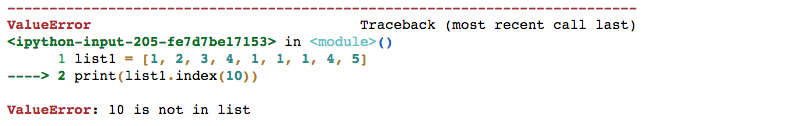```list1 = [1, 2, 3, 4, 1, 1, 1, 4, 5]
print(list1.index(4))```

3

You can use the function listdir from os library in python to achieve the same.

```import os
os.listdir()```

['.ipynb_checkpoints',
'Capture.PNG',
'cars.csv',
'foo.pdf',
'Pandas numpy matplotlib seaborn.ipynb']

Both pivot_table and groupby are used to aggregate your dataframe. The difference is only based on the shape of the result.

`df = pd.DataFrame({"a": [1,2,3,1,2,3], "b":[1,1,1,2,2,2], "c":np.random.rand(6)}) `
`pvt_tbl = pd.pivot_table(df, index=["a"], columns=["b"], values=["c"], aggfunc=np.sum) `
`pvt_tbl`
`df.groupby(['a','b'])['c'].sum()`
• loc gets rows (or columns) with particular labels from the index
• iloc gets rows (or columns) at particular positions in the index (so it only takes integers)
```s = pd.Series(np.nan, index=[49,48,47,46,45, 1, 2, 3, 4, 5])
s.iloc[:3] # slice the first three row i.e. indexes 0,1,2
s.loc[:3] # slice up to and including index label 3```
• apply() is used to Apply a function along an axis (across rows or columns) of the DataFrame and
• applymap() is used to apply a function to a Dataframe elementwise

```df = pd.DataFrame([[4, 9],] * 3, columns=['A', 'B'])
df.apply(np.sum, axis=0)
df.apply(np.sum, axis=1)
f = lambda x: x + 2
df.applymap(f)```
```cars = pd.read_csv('cars.csv')
f = lambda x: "low hp" if x< 100 else "high hp"
cars['hp'] = cars.Horsepower.apply(f)

A Matplotlib plot can be divided into following parts

• Figure

The whole figure. The figure keeps track of all the child Axes, a smattering of ‘special’ artists (titles, figure legends, etc), and the canvas.A figure can have any number of Axes, but to be useful should have at least one.

• Axes

This is what you think of as ‘a plot’, it is the region of the image with the data space (marked as the inner blue box). A given figure can contain many Axes, but a given Axes object can only be in one Figure. The Axes contains two (or three in the case of 3D) Axis objects (be aware of the difference between Axes and Axis) which take care of the data limits (the data limits can also be controlled via set via the set_xlim() and set_ylim() Axes methods). Each Axes has a title (set via set_title()), an x-label (set via set_xlabel()), and a y-label set via set_ylabel()).

• Axis

These are the number-line-like objects (circled in green). They take care of setting the graph limits and generating the ticks (the marks on the axis) and ticklabels (strings labeling the ticks). The location of the ticks is determined by a Locator object and the ticklabel strings are formatted by a Formatter. The combination of the correct Locator and Formatter gives very fine control over the tick locations and labels.

• Artist

Basically everything you can see on the figure is an artist (even the Figure, Axes, and Axis objects). This includes Text objects, Line2D objects, collection objects, Patch objects ... (you get the idea). When the figure is rendered, all of the artists are drawn to the canvas. Most Artists are tied to an Axes; such an Artist cannot be shared by multiple Axes, or moved from one to another.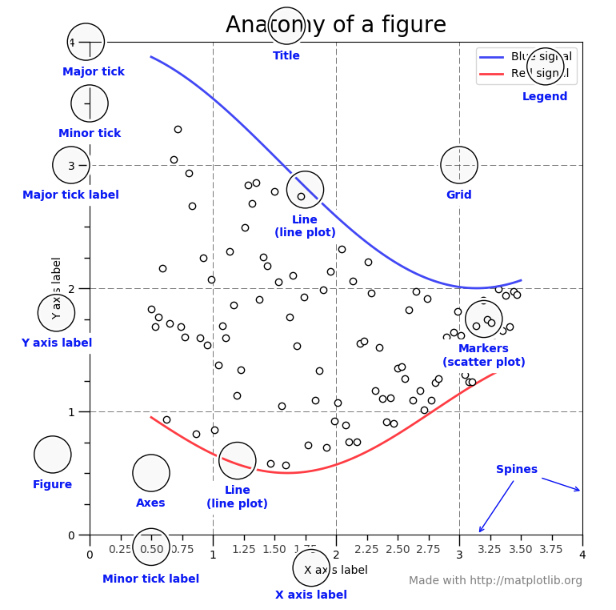Subplots are grid of plots within a single figure. Subplots can be plotted using subplots() function from matplotlib.pyplot module.

```x = np.linspace(0, 2 * np.pi, 400)
y = np.sin(x ** 2)```

## one figure with one subplot

```fig, ax = plt.subplots(ncols=1,nrows=1)
ax.plot(x, y)
plt.plot()```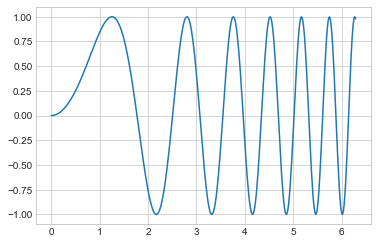```fig1, ax1 = plt.subplots()
ax1.hist(cars.Horsepower)
plt.show()```• remove() removes the first matching value in a given list
• del() removes the item at a specific index
• pop() removes the item at a specific index and returns it.
```# remove() removes the first matching value, not a specific index:
a = [0, 2, 3, 2]
a.remove(2)
a```

[0, 3, 2]

```[0, 3, 2]
a = [3, 2, 2, 1]
del a
a```

[3, 2, 2]

```[3, 2, 2]
a = [4, 3, 5]
a.pop(1)
a```
`[4, 5]`

[4, 5]

• append() adds its argument as a single element to the end of a list. The length of the list itself will increase by one
• extend() iterates over its argument adding each element to the list, extending the list. The length of the list will increase by however many elements were in the iterable argument
```x = ["1", "2", "3","new","old"]
x.extend([4, 5])
print (x
print("Length of list is :",len(x))```

['1', '2', '3', 'new', 'old', 4, 5]
Length of list is : 7

```x = ["1", "2", "3","new","old"]
x.append([4, 5])
print (x)
print("Length of list is :",len(x))```

['1', '2', '3', 'new', 'old', [4, 5]]
Length of list is : 6

```f = plt.figure()
plt.plot(range(10), range(10), "o")
plt.show()```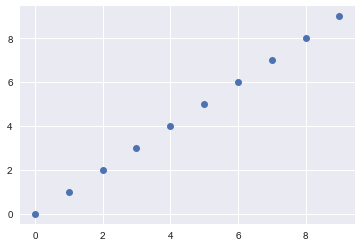```f.savefig("foo.pdf")
```
`cars.head()`
`type(cars)`

pandas.core.frame.DataFrame

`cars.dtypes`

Car                   object
MPG                 float64
Cylinders           int64
Displacement    float64
Horsepower       int64
Weight               int64
Acceleration      float64
Model                int64
Origin                object
hp                     object
dtype:object

```fig, axarr = plt.subplots(2, sharex=True, sharey=True)
axarr.plot(x, y)
axarr.set_title('Subplot 1')
axarr.scatter(x, y)
axarr.set_title('Subplot 2')```

The term broadcasting refers to the ability of NumPy to treat arrays of different shapes during arithmetic operations. If the dimensions of two arrays are dissimilar, element-to-element operations are not possible. However, operations on arrays of non-similar shapes is still possible in NumPy, because of the broadcasting capability. The smaller array is broadcast to the size of the larger array so that they have compatible shapes. NumPy’s broadcasting rule relaxes this constraint when the arrays’ shapes meet certain constraints.

```a = np.array([1.0, 2.0, 3.0])
b = np.array([2.0])
a * b```

array([2., 4., 6.])

When operating on two arrays, NumPy compares their shapes element-wise. It starts with the trailing dimensions, and works its way forward. Two dimensions are compatible when

1. they are equal, or
2. one of them is 1 If these conditions are not met, a ValueError: frames are not aligned exception is thrown, indicating that the arrays have incompatible shapes. The size of the resulting array is the maximum size along each dimension of the input arrays.
```a = np.array([1.0, 2.0, 3.0])
b = np.array([5.0, 2.0])
a * b```

The above line of code will generate 1 figure with 3 subplots arranged in 3 rows and one column with shared x axis.

```fig, axarr = plt.subplots(3, sharex=True, sharey=True)
fig.suptitle('Sharing both axes')
axarr.plot(x, y)
axarr.scatter(x, y)
axarr.scatter(x, 2 * y ** 2 - 1, color='r')```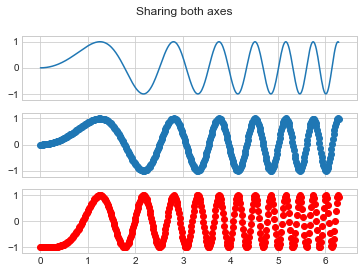When we want a good representation of the distribution of values in data we use swarmplot() from seaborn library in python. But refrain from using swarmplot in case you have large number of observations since it does not scale well to large numbers of observations.

```import matplotlib.pyplot as plt
import seaborn as sns
sns.set_style("whitegrid")```
```tips = sns.load_dataset("tips")
ax = sns.swarmplot(x="day", y="total_bill", data=tips)
ax = sns.boxplot(x="day", y="total_bill", data=tips,
showcaps=False,boxprops={'facecolor':'None'},
showfliers=False,whiskerprops={'linewidth':0})

plt.show()```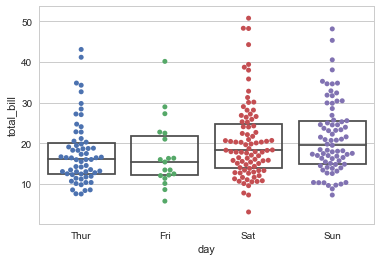Pickling is a process of converting a python object into a byte stream in order to store it in a file/database or to transport data over the network. Pickle module accepts any Python object and converts it into a string representation and dumps it into a file by using dump function, this process is called pickling. While the process of retrieving original Python objects from the stored string representation is called unpickling.

```import pickle

with open('filename', 'wb') as f:
var = {1 : 'a' , 2 : 'b'}
pickle.dump(var, f)
#That would store the pickled version of our var dict in the 'filename' file in your home directory.

#Then, in another script, you could load from this file into a variable and the dictionary would be recreated:
with open('filename','rb') as f:```
`var = pickle.load(f) `
```You could use pickle library and use the following:
dump(model,"file") ```

A sparse matrix can be generated by using rand function in scipy.sparse module.
rand(m, n, density=0.01, format='coo', dtype=None, random_state=None)

• m, n : int shape of the matrix
• density : real, optional density of the generated matrix: density equal to one means a full matrix, density of 0 means a matrix with no non-zero items
• format : str, optional sparse matrix format
• dtype : dtype, optional type of the returned matrix values
• random_state : {numpy.random.RandomState, int}, optional Random number generator or random seed. If not given, the singleton numpy.random will be used
```from scipy.sparse import rand
matrix = rand(3, 4, density=0.25, format="dense", random_state=42)
matrix```
```X = np.linspace(0,5,100)
Y1 = X + 2*np.random.random(X.shape)
Y2 = X**2 + np.random.random(X.shape)```
`fig1, ax1 = plt.subplots() `
```ax1.scatter(X,Y1,color='k')
ax1.plot(X,Y2,color='g')
plt.show()```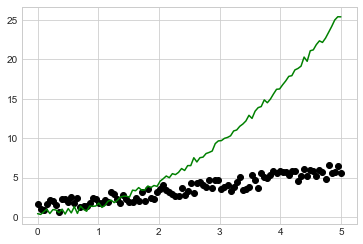`cars.head()`
```fig1, ax1 = plt.subplots()
ax1.scatter(cars.Horsepower,cars.Displacement,c=cars.Acceleration)
plt.show()```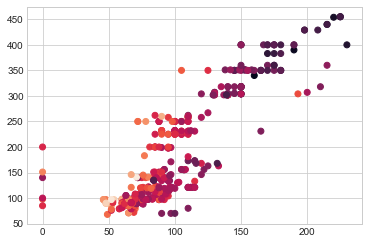`help(pd.Series.loc)`

Violin plots allow to visualize the distribution of a numeric variable for one or several groups. It is really close from a boxplot, but allows a deeper understanding of the density.

```df = pd.DataFrame(
{"Purchase": 50 * ["Yes"] + 50 * ["No"],
"A": np.random.randint(1, 7, 100),
"B": np.random.randint(1, 7, 100)}
)
import seaborn as sns
sns.violinplot(data=df[["A", "B"]], inner="quartile", bw=.15)```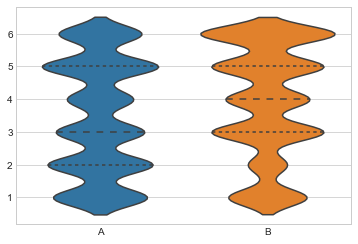list.sort() sorts the list and save the sorted list, while sorted(list) returns a sorted copy of the list, without changing the original list. sorted() returns a new sorted list, leaving the original list unaffected. list.sort() sorts the list in-place, mutating the list indices, and returns None (like all in-place operations). sorted() works on any iterable, not just lists. Strings, tuples, dictionaries (you'll get the keys), generators, etc., returning a list containing all elements, sorted.

When to use sort() and when to use sorted()?

• Use list.sort() when you want to mutate the list, sorted() when you want a new sorted object back

Which is fast to use for lists - sort() or sorted()?

• For lists, list.sort() is faster than sorted() because it doesn't have to create a copy. For any other iterable, you have no choice

Can a list's original positions be retrieved after list.sort()?

• No, you cannot retrieve the original positions. Once you called list.sort() the original order is gone
```a = [3, 2, 1]

a2 = sorted(a)
print (a, a2)```

[3, 2, 1] [1, 2, 3]

```a3 = a.sort()
print (a, a3)```

[1, 2, 3] None

This function combines the matplotlib hist function (with automatic calculation of a good default bin size) with the seaborn kdeplot() and rugplot() functions. It can also fit scipy.stats distributions and plot the estimated PDF over the data.

```import seaborn as sns
sns.set(); np.random.seed(0)
x = np.random.randn(100)
ax = sns.distplot(x)```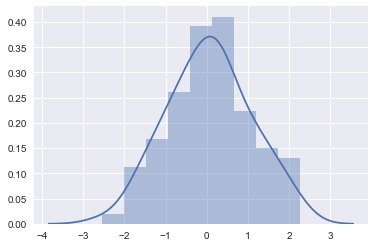`np.vstack(([1,2,3],[4,5,6]))`

array([[1, 2, 3],
[4, 5, 6]])

`np.column_stack(([1,2,3],[4,5,6]))`

array([[1, 4],
[2, 5],
[3, 6]])

`np.hstack(([1,2,3],[4,5,6]))`

array([1, 2, 3, 4, 5, 6])

`np.hstack(([,,],[,,]))`

array([[1, 4],
[2, 5],
[3, 6]])

Pandas DataFrame object has a method astype() to cast to a specified dtype dtype.

`cars.MPG[0:5]`

0    18.0
1    15.0
2    18.0
3    16.0
4    17.0
Name: MPG, dtype: float64

`cars.MPG = cars.MPG.astype('int')`
`cars.dtypes`

Car                 object
MPG               int64
Cylinders        int64
Displacement float64
Horsepower   int64
Weight           int64
Acceleration  float64
Model            int64
Origin            object
hp                 object
dtype: object

`cars`
`cars.sort_index(axis=1,ascending=True).head(5)`
`cars.head()`
`cars.rename(columns={'Horsepower':'horse_power'},inplace=True)`
`cars.head()`
`cars.loc[cars.Car.isin(['AMC Hornet','Volkswagen Rabbit'])]`
`x = cars.Car.value_counts() # 'Chevrolet Impala'`
`x[x.index == 'Chevrolet Impala']`

Chevrolet Impala    4
Name: Car,  dtype:  int64

Dash is a Python framework for building analytical web applications by Plotly.To build web applications using Dash we don't need JavaScript.

Describe() function Generates descriptive statistics that summarize the central tendency, dispersion and shape of a dataset’s distribution, excluding NaN values.

`cars.describe() `
`cars.loc[cars.Car.isin(['AMC Hornet','Volkswagen Rabbit'])] `

Pandas library provides read_csv() function to read a csv file. To skip first three rows we need to pass a value to argument skiprows to read_csv() function. skiprows can be list or integer.Line numbers to skip (0-indexed) or number of lines to skip (int) at the start of the file.

`pd.read_csv('cars.csv',skiprows=3)`

The lambda operator or lambda function is a way to create small anonymous functions, i.e. functions without a name.Basic syntax of a lambda function is

. lambda argument list : expression.

The arguments list consists of a comma separated list of arguments and the expression is an arithmetic expression using these arguments.

In add =lambda x, y: x + y; x and y are arguments to the function and x + y is the expression which gets executed and its values is returned as output. lambda x, y: x + y returns a function object which can be assigned to any variable, in this case function object is assigned to the add variable. Mostly lambda functions are passed as parameters to a function which expects a function objects as parameter like map, reduce, filter functions.

```add = lambda x, y : x + y

3

Seaborn provides five preset themes: white grid, dark grid, white, dark, and ticks, each suited to different applications and also personal preferences. Darkgrid is the default one. The White grid theme is similar but better suited to plots with heavy data elements, to switch to white grid.

```sns.set_style("whitegrid")
data = np.random.normal(size=(20, 6)) + np.arange(6) / 2
sns.boxplot(data=data)```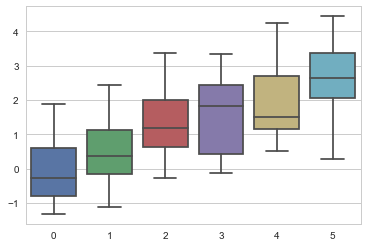```sns.set_style("darkgrid")
data = np.random.normal(size=(20, 6)) + np.arange(6) / 2
sns.boxplot(data=data)```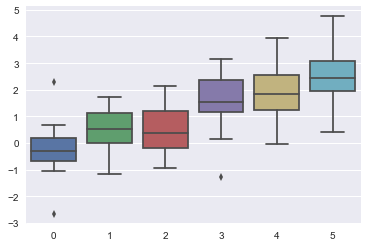```Map applies a function to all the items in an input list
map(function object, list1, list2,...) map functions expects a function object and any number of iterables.It executes the function over lists and returns a map object.

list(map(lambda x : x*x, [1, 2, 3, 10])) ```
```[1, 4, 9, 100]

list(map(lambda x,y : x*y, [1, 2, 3, 10],[2,2,2,2]))```

[2, 4, 6, 20]

List comprehensions provide a concise way to create lists. Common applications are to make new lists where each element is the result of some operations applied to each member of another sequence or iterable, or to create a subsequence of those elements that satisfy a certain condition.A list comprehension always returns a result list. Basic syntax : new list = [expression(i) for i in old list if filter(i)]

` [x**2 for x in range(10)] `

[0, 1, 4, 9, 16, 25, 36, 49, 64, 81]

np.mean() Computes the arithmetic mean along the specified axis. It Returns the average of the array elements. The average is taken over the flattened array by default, otherwise over the specified axis. float64 intermediate and return values are used for integer inputs

`a = np.array([[1, 2], [3, 4]]) `
`np.mean(a) `

2.5

`np.mean(a, axis=0)`

array([2., 3.])

`np.mean(a, axis=1)`

array([1.5, 3.5])

Use subplots_adjust.When using subplots_adjust, the values of left, right, bottom and top are to be provided as fractions of the figure width and height. In additions, all values are measured from the left and bottom edges of the figure. This is why right and top can't be lower than left and bottom.

```plt.subplots_adjust(left=0.1, right=0.9, bottom=0.1, top=0.9)
fig = plt.figure()
```for i in range(1, 7):
ax = fig.add_subplot(2, 3, i)
ax.text(0.5, 0.5, str((2, 3, i)),
fontsize=18, ha='center')```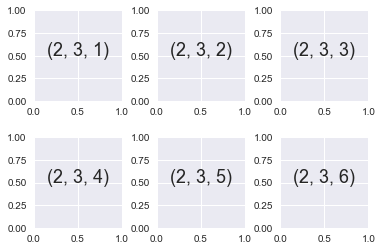Flask is a web micro framework for Python based on "Werkzeug, Jinja 2 and good intentions" BSD licensed and build for a small application with simpler requirements Werkzeug and Jinja are two of its dependencies. Flask is part of the micro-framework. Which means it will have little to no dependencies on external libraries. It mistakes the framework light while there is little dependency to update and less security bugs.

This plot lets you easily view both a joint distribution and its marginals at once. Joint distribution plots combine information from scatter plots and histograms to give you detailed information for bivariate distributions.

```x, y = np.random.RandomState(8).multivariate_normal([0, 0], [(1, 0), (0, 1)], 1000).T

```
```df = pd.DataFrame({"x":x,"y":y})
p = sns.jointplot(data=df,x='x', y='y')
# kde plots a kernel density estimate in the margins and converts the interior into a shaded countour plot
p = sns.jointplot(data=df,x='x', y='y',kind='kde')```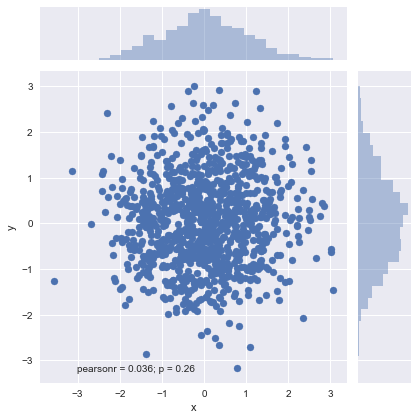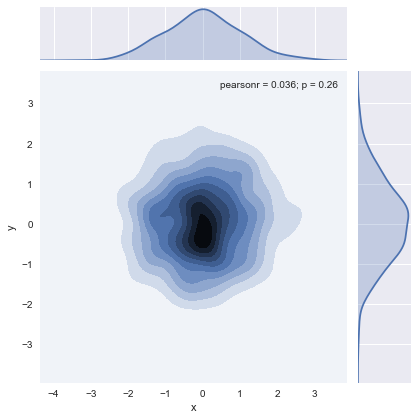Split() is used to break a large string down into smaller chunks, or strings. If no separator is defined when you call upon the function, whitespace will be used by default. In simpler terms, the separator is a defined character that will be placed between each variable.

```x = "blue,red,green"
x.split(",")```

['blue', 'red', 'green']

```# Create data
data = {'score': [1,1,1,2,2,2,3,3,3]}
# Create dataframe
df = pd.DataFrame(data)
# View dataframe
df```
```# Calculate the moving average. That is, take the first two values, average them, then drop the first and add the third, etc.
print(df)```

score
0      1
1      1
2      1
3      2
4      2
5      2
6      3
7      3
8      3

`print("ROLLING MEAN VALUES ARE:",df.rolling(window=2).mean())`

ROLLING MEAN VALUES ARE:    score
0    NaN
1    1.0
2    1.0
3    1.5
4    2.0
5    2.0
6    2.5
7    3.0
8    3.0

```df = sns.load_dataset('iris')
sns.regplot(x=df["sepal_length"], y=df["sepal_width"], fit_reg=False)```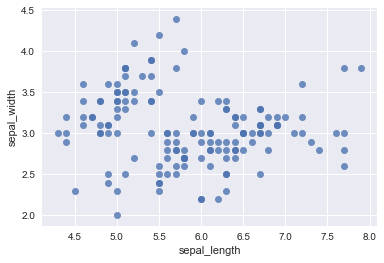```uniform_data = np.random.rand(10, 12)
ax = sns.heatmap(uniform_data)```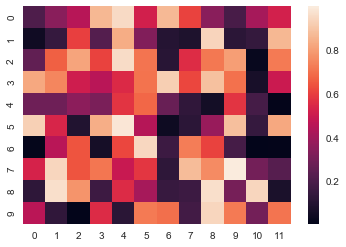`pd.read_excel('tmp.xlsx') `

range() – This returns a list of numbers created using range() function.
xrange() – This function returns the generator object that can be used to display numbers only by looping. Only particular range is displayed on demand and hence called lazy evaluation. In Python 3, they removed the original range function and renamed xrange to a range.

To modify the strings, Python “re” module is providing 3 methods.

split() - uses a regex pattern to “split” a given string into a list.

sub() - finds all substrings where the regex pattern matches and then replace them with a different string

subn() - it is similar to sub() and also returns the new string along with the no. of replacements.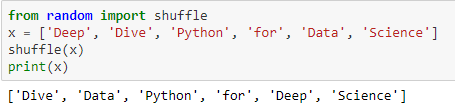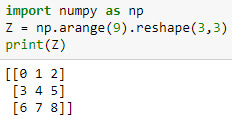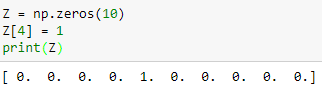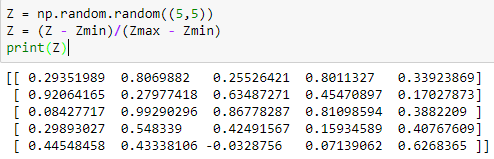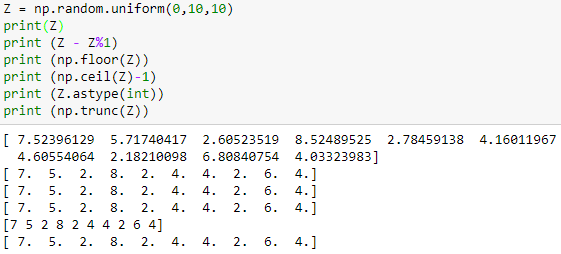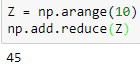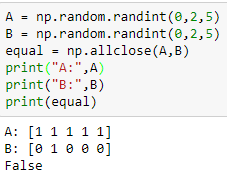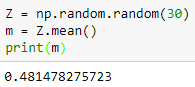A[1, 4] access the data item at the 5th column, 2nd row.

readlines() : Reads all the lines and return each line as a string element in a list and readline() : Reads a line of the file and returns in form of a string. For specified n, reads at most n bytes. However, does not read more than one line, even if n exceeds the length of the line.

True. ndarray.dataitemSize is the buffer containing the actual elements of the array.

np.eye(3) whereas, library numpy has been imported as np.

`df.to_csv(‘/file.csv’,encoding=’utf-8′,index=False,header=False).`

def MSE(real_target, predicted_target):
return np.mean((real_target – predicted_target)**2)

A tuple cannot be updated. Tuples are immutable. This means that you cannot change the values in a tuple once you have created it.

urllib2.urlopen(www.pythondeepdive.org) and requests.get(www.pythondeepdive.org) are the two forms to read the website.

set_index(‘String’)[‘Count’].to_dict()

You can put bookmark as time.sleep() so that you would know how much the code has slept,  put bookmark as time.time() and check how much time elapses in each code line and copy whole code in an Ipython/Jupyter notebook, with each code line as a separate block and write function %%timeit in each block.

a.extend(b) function would do the job of converting it to one dimension.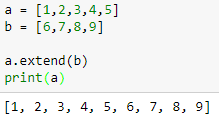An OrderedDict is a dictionary subclass that remembers the order in which its contents are added. If a new entry overwrites an existing entry, the original insertion position is left unchanged. Deleting an entry and reinserting it will move it to the end.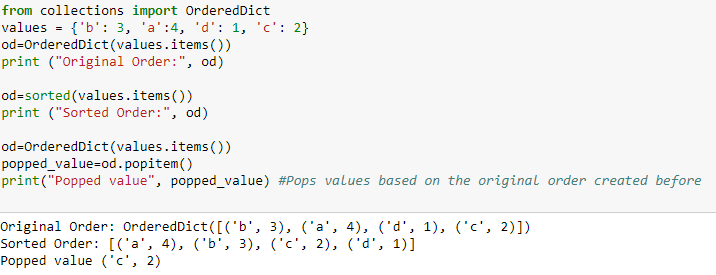indall() function is used to return all the non-overlapping matches of patterns in the string as the list of strings.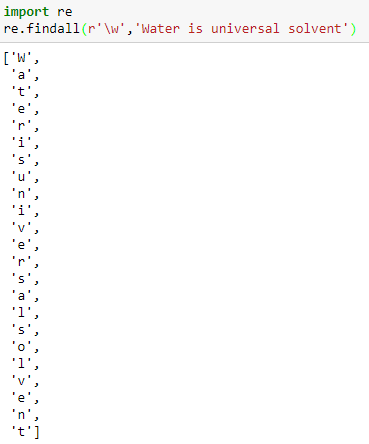The partition method returns a 3-tuple containing:

• the part before the separator, separator parameter, and the part after the separator if the separator parameter is found in the string
• string itself and two empty strings if the separator parameter is not found

Here, the entire string has been passed as the separator hence the first and the last item of the tuple returned are null strings.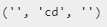3 defines the precision of the floating point number.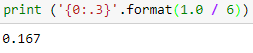• Pandas: Pandas is an open-source Python Library providing high-performance data manipulation and analysis tool using its powerful data structures
• NumPy: NumPy enriches the programming language Python with powerful data structures, implementing multi-dimensional arrays, and matrices. These data structures guarantee efficient calculations with matrices and arrays
• Matplotlib: A 2D rendering engine written for Python
• TensorFlow: A package used for constructing computational graphs. Neural network sand many machine learning models depend on these computational graphs

2 is the view of original dataframe and 1 is a copy of original dataframe.

## Description

Prepare better with the best interview questions and answers, and walk away with top interview tips. These interview questions and answers will boost your core interview skills and help you perform better. Be smarter with every interview.